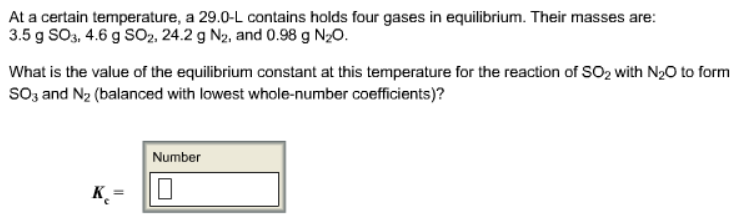# Problem: At a certain temperature, a 29.0-L contains holds four gases in equilibrium. Their masses are: 3.5 g SO3, 4.6 g SO2, 24.2 g N2, and 0.98 g N2O. What is the value of the equilibrium constant at this temperature for the reaction of SO2 with N2O to form SO3 and N2 (balanced with lowest whole-number coefficients)?

###### FREE Expert Solution
98% (371 ratings)###### Problem Details

At a certain temperature, a 29.0-L contains holds four gases in equilibrium. Their masses are: 3.5 g SO3, 4.6 g SO2, 24.2 g N2, and 0.98 g N2O.

What is the value of the equilibrium constant at this temperature for the reaction of SO2 with N2O to form SO3 and N2 (balanced with lowest whole-number coefficients)?